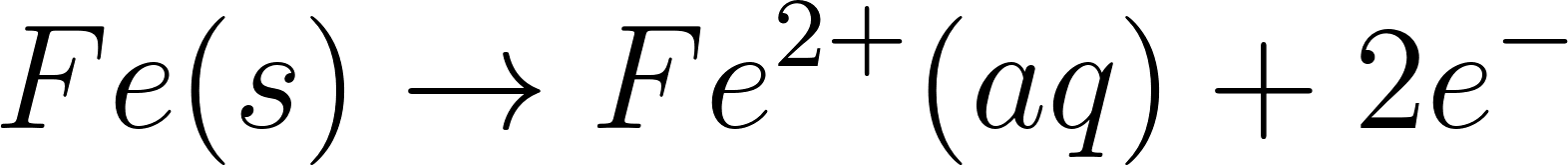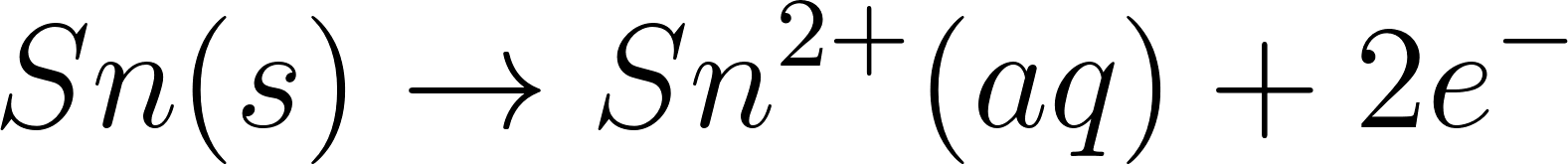×
Get Full Access to Introductory Chemistry - 5 Edition - Chapter 16 - Problem 72p
Get Full Access to Introductory Chemistry - 5 Edition - Chapter 16 - Problem 72p

×

# Which metal has the least tendency to be oxidized?(a) Sn(b) Mg(c) Cu(d) FeISBN: 9780321910295 34

## Solution for problem 72P Chapter 16

Introductory Chemistry | 5th Edition

• Textbook Solutions
• 2901 Step-by-step solutions solved by professors and subject experts
• Get 24/7 help from StudySoup virtual teaching assistantsIntroductory Chemistry | 5th Edition

4 5 1 420 Reviews
18
3
Problem 72P

Problem 72P

Which metal has the least tendency to be oxidized?

(a)  Sn

(b) Mg

(c) Cu

(d) Fe

Step-by-Step Solution:

Problem 72P

Which metal has the least tendency to be oxidized?

(a)  Sn

(b) Mg

(c) Cu

(d) Fe

Step by step solution

Step 1 of 2

Let’s write the oxidation state of each element.Step 2 of 2

## Discover and learn what students are asking

Calculus: Early Transcendental Functions : Inverse Trigonometric Functions: Integration
?In Exercises 1-20, find the indefinite integral. $$\int \frac{1}{x \sqrt{4 x^{2}-1}} d x$$

Statistics: Informed Decisions Using Data : Inference on the Least-Squares Regression Model and Multiple Regression
?NFL Combine Download the data file 14_cr_10.txt at www.pearsonhighered.com/sullivanstats. The data represent a variety of variables measured on player

Unlock Textbook Solution